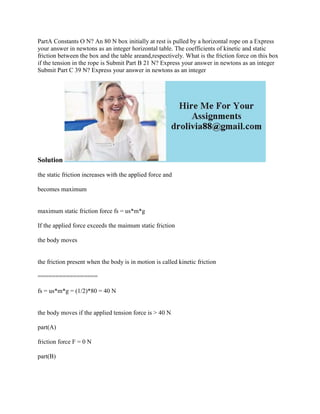Seu SlideShare está sendo baixado. ×

# PartA Constants O N- An 80 N box initially at rest is pulled by a hori.docx

Anúncio
Anúncio
Anúncio
Anúncio
Anúncio
Anúncio
Anúncio
Anúncio
Anúncio
Anúncio
AnúncioCarregando em…3
×

1 de 2 Anúncio

# PartA Constants O N- An 80 N box initially at rest is pulled by a hori.docx

PartA Constants O N? An 80 N box initially at rest is pulled by a horizontal rope on a Express your answer in newtons as an integer horizontal table. The coefficients of kinetic and static friction between the box and the table areand,respectively. What is the friction force on this box if the tension in the rope is Submit Part B 21 N? Express your answer in newtons as an integer Submit Part C 39 N? Express your answer in newtons as an integer
Solution
the static friction increases with the applied force and
becomes maximum
maximum static friction force fs = us*m*g
If the applied force exceeds the maimum static friction
the body moves
the friction present when the body is in motion is called kinetic friction
=================
fs = us*m*g = (1/2)*80 = 40 N
the body moves if the applied tension force is > 40 N
part(A)
friction force F = 0 N
part(B)
friction force F = 21 N
part ( c )
friction force F = 39 N
part(D)
friction force F = uk*m*g = (1/4)*80 = 20 N
part(E)
friction force F = uk*m*g = (1/4)*80 = 20 N
.

PartA Constants O N? An 80 N box initially at rest is pulled by a horizontal rope on a Express your answer in newtons as an integer horizontal table. The coefficients of kinetic and static friction between the box and the table areand,respectively. What is the friction force on this box if the tension in the rope is Submit Part B 21 N? Express your answer in newtons as an integer Submit Part C 39 N? Express your answer in newtons as an integer
Solution
the static friction increases with the applied force and
becomes maximum
maximum static friction force fs = us*m*g
If the applied force exceeds the maimum static friction
the body moves
the friction present when the body is in motion is called kinetic friction
=================
fs = us*m*g = (1/2)*80 = 40 N
the body moves if the applied tension force is > 40 N
part(A)
friction force F = 0 N
part(B)
friction force F = 21 N
part ( c )
friction force F = 39 N
part(D)
friction force F = uk*m*g = (1/4)*80 = 20 N
part(E)
friction force F = uk*m*g = (1/4)*80 = 20 N